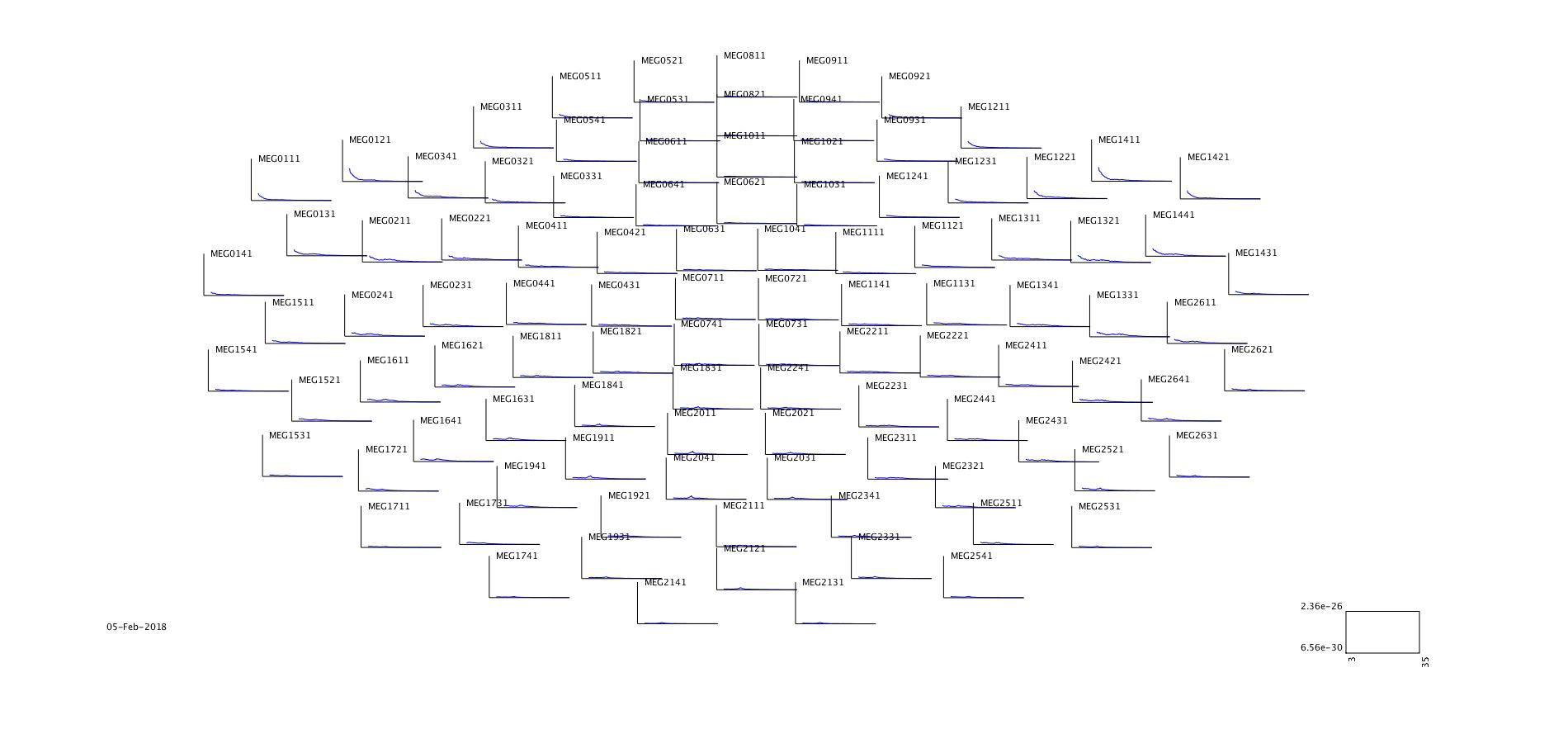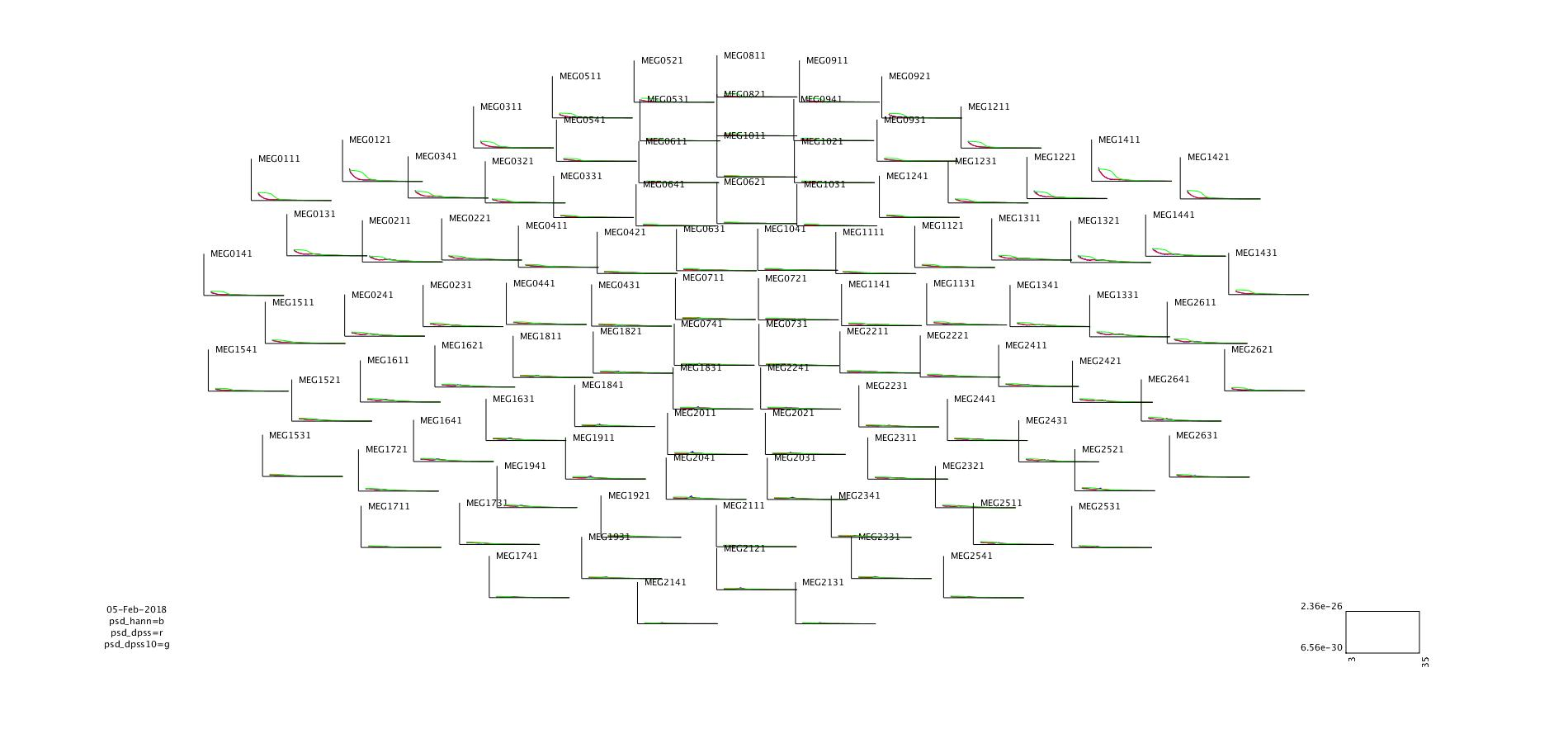Set up general paths

Change these to the appropriate paths for your operating system and setup.

ft_defaults

raw_meg_path = '/archive/20067_workshop_source_reconstruction/MEG/';
meg_path = '/home/share/workshop_source_reconstruction/data/MEG/';
mri_path = '/home/share/workshop_source_reconstruction/data/MRI/';

%% subjects and dates
subjects_and_dates = ...
{
'NatMEG_0177/170424/'
};

data_path = fullfile(meg_path, subjects_and_dates{1});
cd(data_path);

Read in cleaned data from yesterday (remember where you put the data and what you named it).

If you did not complete the data preperation tutorial, you can load the data file cleaned_downsampled_data.mat from the tutorial material:

disp('Done');

In the following tutorial, we will only analyse the conditions where the index finger was stimulated, i.e. the condition corresponding to trigger value 8. Use ft_selectdata to select the conditions with trigger 8:

cfg = [];
cfg.trials = cleaned_downsampled_data.trialinfo==8;

epochs = ft_selectdata(cfg, cleaned_downsampled_data)

First, we baseline correct the data that we are going to use for the TFRs - i.e. demean the epochs.

cfg = [];
cfg.demean          = 'yes';
cfg.baselinewindow  = 'all';

epochs_bs = ft_preprocessing(cfg, epochs);

Estimate Power Spectral Density

We are going to compute the power spectral density (PSD) across the epochs. This will give us the average power across all trials. We will use different methods to estimate PDS: 1) a single taper window, and 2) multi-tapered windows. All calculations will be done with the FieldTrip function ft_freqanalysis. ft_freqanalysis is the default function for running various types of frequency analysis such as calculating PSD and doing the time-frequency analysis. To get more info type:

help ft_freqanalysis

Get PSD with single taper

In the first calculation, we will use a Hann window to taper the epochs. This is applied to each epoch in the data structure before doing Fourier decomposition and then calculating the power. The Hann window is selected by passing the configuration cfg.taper = ‘hanning’ to ft_freqanalysis.

NB. If the process is slow, change cfg.channel to ’MEG*1’ to select only MEG magnetometers to run. You can always come back and re-run the analysis.

cfg = [];
cfg.output      = 'pow';          % Return PSD
cfg.channel     = {'MEG','EEG'};  % Calculate for MEG and EEG
cfg.method      = 'mtmfft';
cfg.taper       = 'hanning';      % Hann window as taper
cfg.foilim      = [1 95];         % Frequency range

psd_hann = ft_freqanalysis(cfg, epochs_bs);

Once finished, look what is in the structure psd_hann.

• Q: What is the dimension of the data, and what do the dimensions represent?

Plot the PSD using ft_multiplotER. the configuration cfg.xlim specifies the limits of the frequency axis.

cfg = [];
cfg.parameter       = 'powspctrm';
cfg.layout          = 'neuromag306mag'; % Layout for MEG magnetometers
cfg.showlabels      = 'yes';
cfg.xlim            = [3 35];           % Frequencies to plot

figure;
ft_multiplotER(cfg, psd_hann);Get PSD with multitapers

Now we will do the same analysis, but use tapers based on Slepian sequences of tapers. The spectral smoothing is specified by passing the frequency range in the configuration cfg.tapsmofrq when calling ft_freqanalysis. From this ft_freqanalysis will calculate the amount of tapers needed to achieve the desired frequency smoothing. Note that the frequency smoothing is the range in either direction, so the frequency smoothing is the double of the numerical value given in _cfg.tapsmofrq.

• Q: How many tapers is used when you run the command below? Hint: it tells you in the terminal.
cfg = [];
cfg.output      = 'pow';          % Return PSD
cfg.channel     = {'MEG','EEG'};  % Calculate for MEG and EEG
cfg.method      = 'mtmfft';
cfg.taper       = 'dpss';         % Multitapers based on Slepian sequences
cfg.tapsmofrq   = 2;              % Smoothing +/- 2 Hz
cfg.foilim      = [1 95];

psd_dpss = ft_freqanalysis(cfg, epochs_bs);

Plot the PSD using ft_multiplotER as above.

Try to change the frequency smoothing range to e.g 10 Hz.

cfg.tapsmofrq   = 10;            % Q: How many tapers does this use?
psd_dpss10 = ft_freqanalysis(cfg, epochs_bs);

Compare the single taper PSD, and the two multitaper PSD you have calculated. Plot them side-by-side using ft_multiplotER

cfg = [];
cfg.parameter       = 'powspctrm';
cfg.layout          = 'neuromag306mag'; % Layout for MEG magnetometers
cfg.showlabels      = 'yes';
cfg.xlim            = [3 35];           % Frequencies to plot

figure;
ft_multiplotER(cfg, psd_hann, psd_dpss, psd_dpss10);Compare the results from the different methods to calculate PSD: Select alpha range (~8-12 Hz) in the multiplot to plot as topoplots.

• Q: How different/alike are they? Explain in your own words why?

Try to select the beta range (~14-30 Hz) and compare topoplots.

• Q: How different/alike are they? Explain in your own words why?

Try to plot the “high-gamma” range (~55-95 Hz) by changing cfg.xlim to [55 95] and call ft_multiplotER_ as before.

• Q: How do the high-gamma spectra compare between methods? Explain in your own words why?

Bonus: Change the layout to view the PSD of EEG

cfg = [];
cfg.parameter   = 'powspctrm';
cfg.layout      = 'neuromag306eeg1005_natmeg.lay'; # EEG layout
cfg.showlabels  = 'yes';
cfg.xlim        = [3 35];
figure;
ft_multiplotER(cfg, psd_hann);

Time-frequency analysis

The PSD analysis above (sort of) assumes that the spectral power is the same across the entire epoch. This is the way we often analyze resting state data or similar paradigms. But we can also analyze how the spectral signals evolve over time, e.g. how oscillatory activity changes as a response to stimuli.

Instead of calculating the power across the entire epoch and then average all epochs, we now will calculate the power for each time sample: We center the window on a given time sample and estimate the power of that particular window. As above, we can choose to taper the window with a single taper (e.g., the Hann window) or by using multitapers. Finally, we will also use Wavelet analysis, which are sequences of tapered sine-waves fitted to the data for the given time point.

Get TFR with single taper MEG

In FieldTrip we calculate the time-frequency response (TFR) with the function ft_freqanalysis, as we did for the PSD. This time we change the method to ‘mtmconvol’. We also have to specify the time sample resolution (toi – times of interest) and the length of the window centered on the time of interest (t_ftimwin – window length).

NB. TFR calculations take significantly longer time to calculate than PSD. You can always change cfg.channel to ’MEG*1’ to save time. You can always go back and redo the other channel types (but do make sure you finish the exercise).

cfg = [];
cfg.output      = 'pow';
cfg.channel     = {'MEG','EEG'};      % Change to 'MEG*1' to analyse all channels
cfg.method      = 'mtmconvol';
cfg.taper       = 'hanning';    % Hann window as taper
cfg.foi         = 1:1:45;       % Frequencies we want to estimate from 1 Hz to 45 Hz in steps of 1HZ
cfg.toi         = -1.650:0.01:1.650;            % Timepoints to center on
cfg.t_ftimwin   = 0.5*ones(length(cfg.foi),1);  % length of time window

tfr_hann = ft_freqanalysis(cfg, epochs_bs);

Plot the result with ft_multiplotTFR:

cfg = [];
cfg.parameter       = 'powspctrm';
cfg.layout          = 'neuromag306mag';
cfg.showlabels      = 'yes';

figure;
ft_multiplotTFR(cfg, tfr_hann);
• Q: This plot look weird! How come? What can we see from the plot (Hint: Remember the PSD plots from before)?